NCERT Exemplar - MCQs

Chapter 5 Class 12 Continuity and Differentiability
Serial order wise

## (D) None of these

This question is similar to Example 43 - Chapter 5 Class 12 - Continuity and Differentiability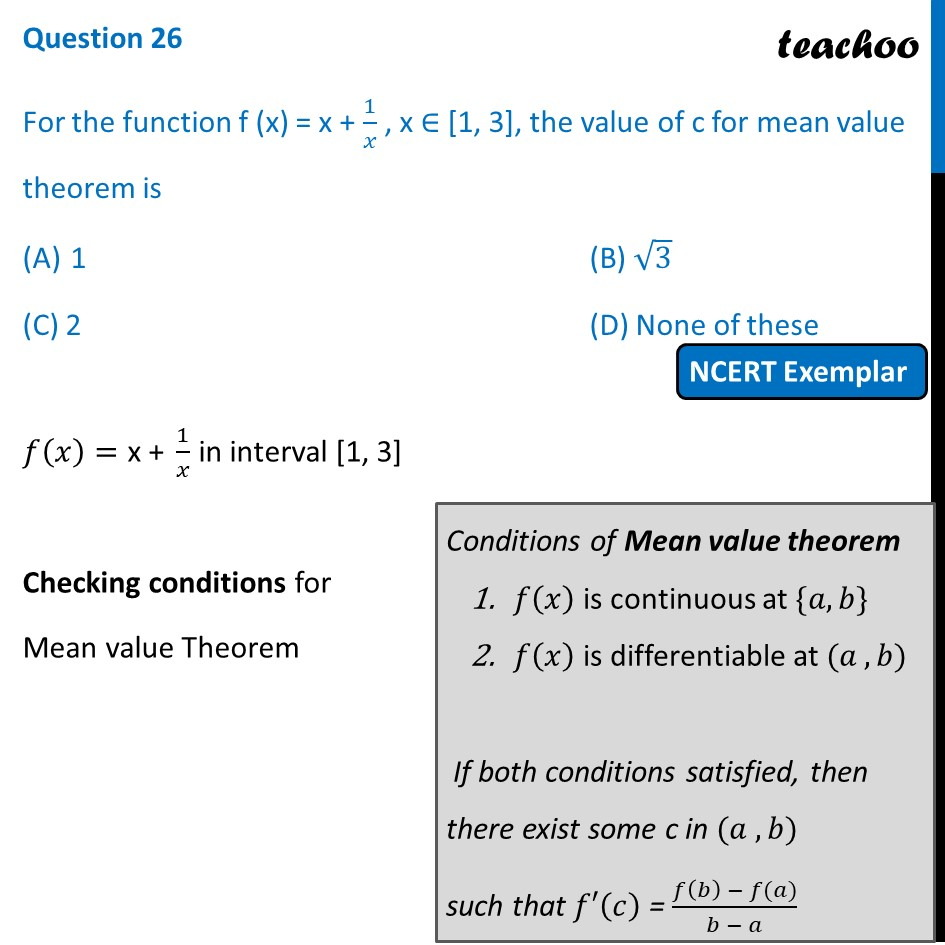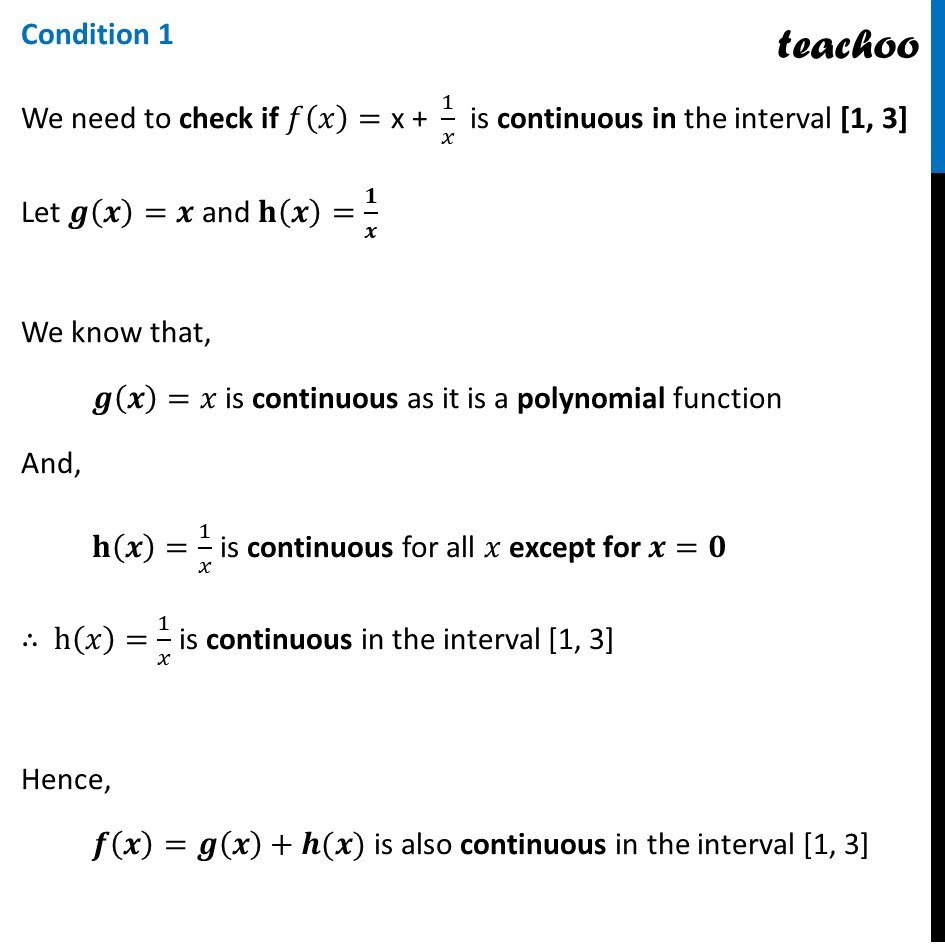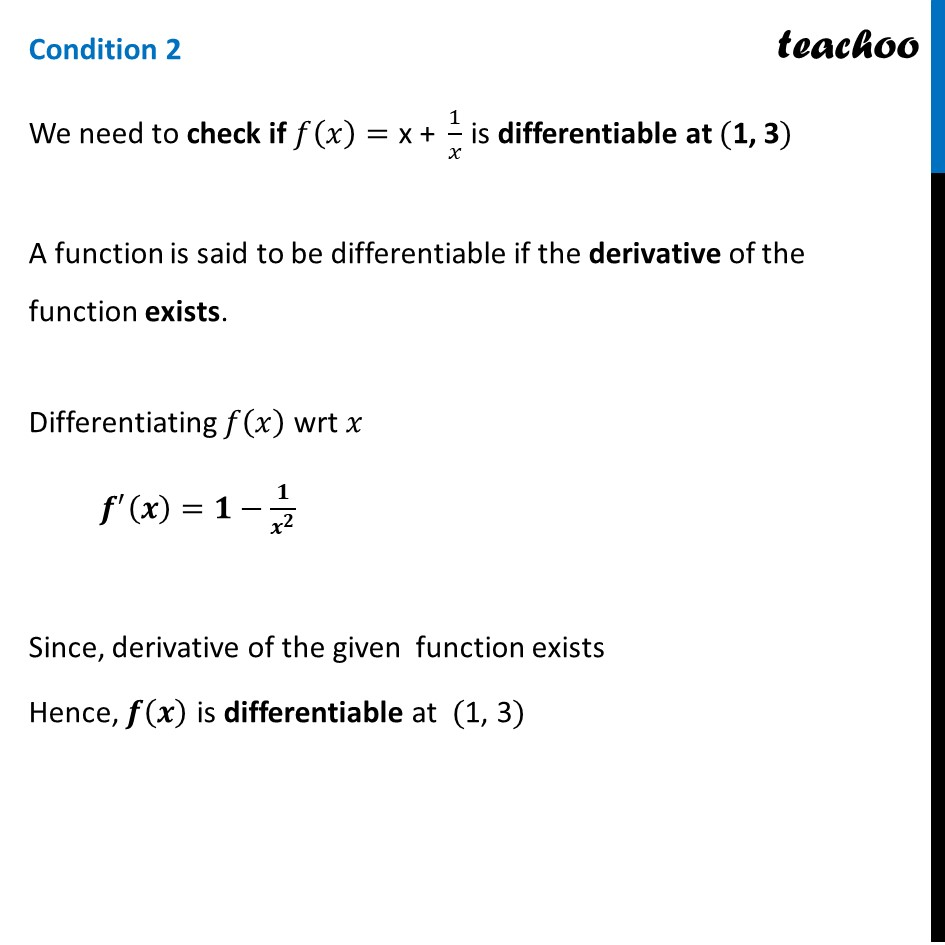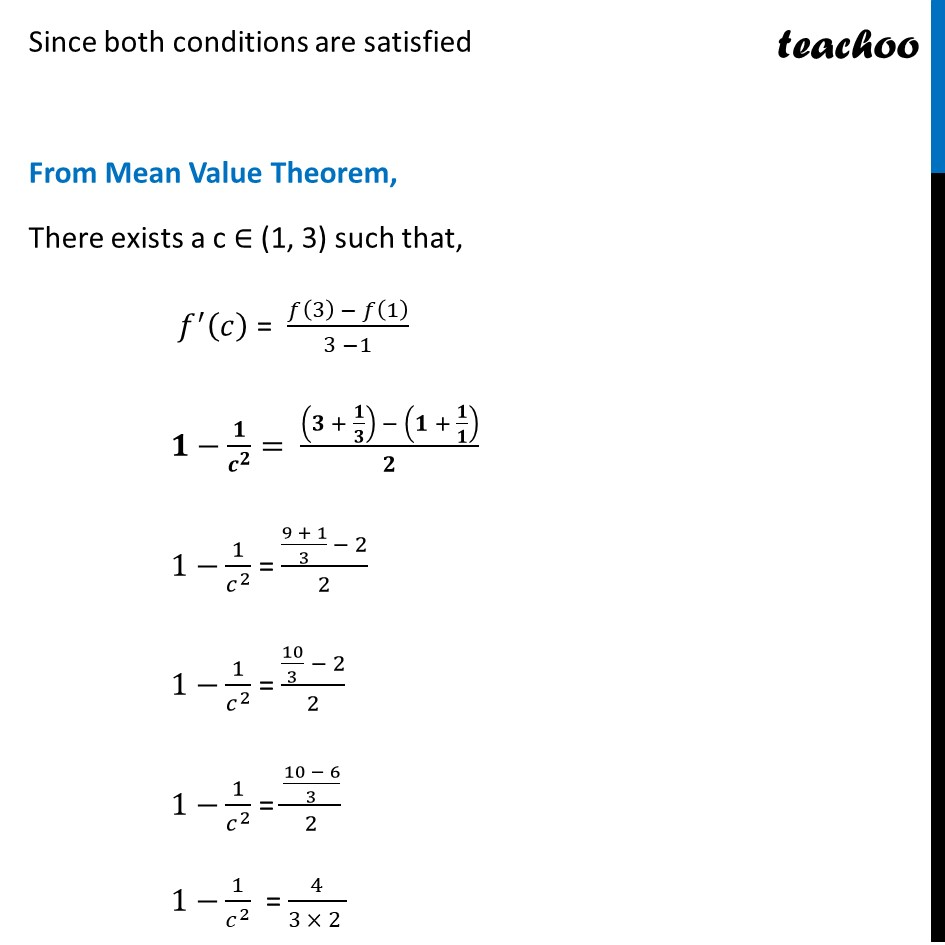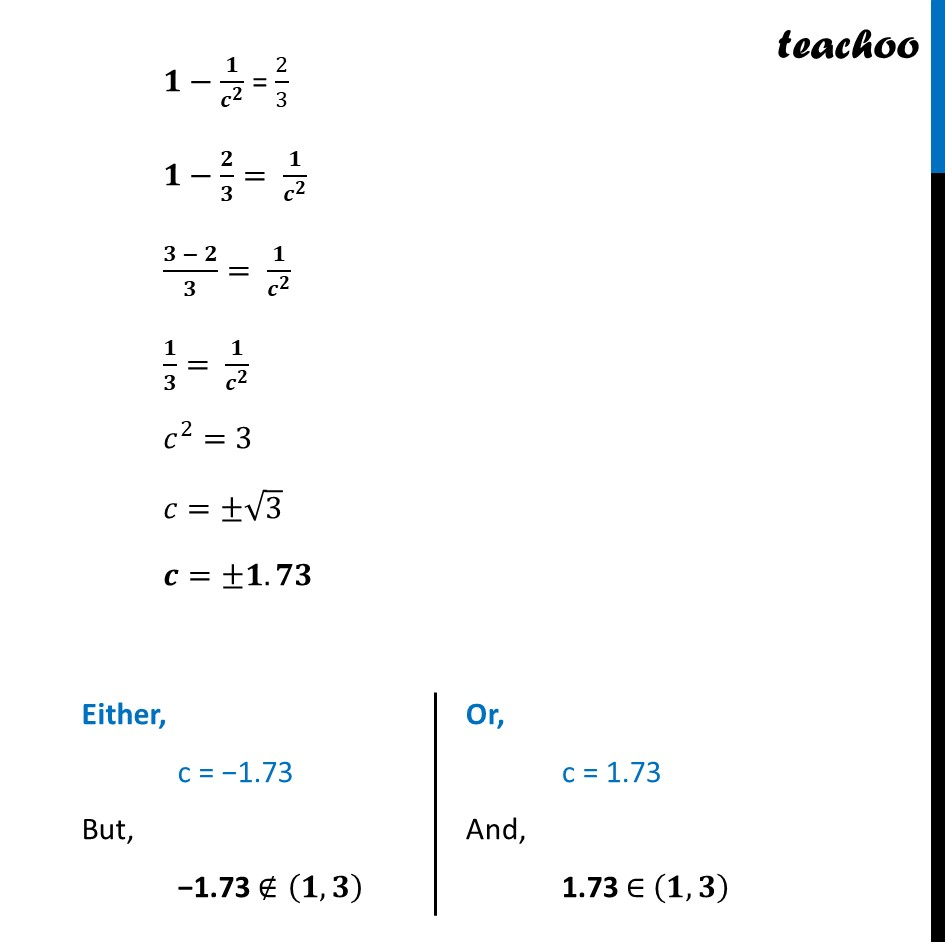Get live Maths 1-on-1 Classs - Class 6 to 12

### Transcript

Question 26 For the function f (x) = x + 1/𝑥 , x ∈ [1, 3], the value of c for mean value theorem is 1 (B) √3 (C) 2 (D) None of these 𝑓(𝑥)="x + " 1/𝑥 in interval [1, 3] Checking conditions for Mean value Theorem Conditions of Mean value theorem 𝑓(𝑥) is continuous at {𝑎, 𝑏} 𝑓(𝑥) is differentiable at (𝑎 , 𝑏) If both conditions satisfied, then there exist some c in (𝑎 , 𝑏) such that 𝑓′(𝑐) = (𝑓(𝑏) − 𝑓(𝑎))/(𝑏 − 𝑎) Condition 1 We need to check if 𝑓(𝑥)="x + " 1/𝑥 is continuous in the interval [1, 3] Let 𝒈(𝒙)=𝒙 and 𝐡(𝒙)=𝟏/𝒙 We know that, 𝒈(𝒙)=𝑥 is continuous as it is a polynomial function And, 𝐡(𝒙)=1/𝑥 is continuous for all 𝑥 except for 𝒙=𝟎 ∴ h(𝑥)=1/𝑥 is continuous in the interval [1, 3] Hence, 𝒇(𝒙)=𝒈(𝒙)+𝒉(𝒙) is also continuous in the interval [1, 3] Condition 2 We need to check if 𝑓(𝑥)="x + " 1/𝑥 is differentiable at ("1, 3") A function is said to be differentiable if the derivative of the function exists. Differentiating 𝑓(𝑥) wrt 𝑥 𝒇^′ (𝒙)=𝟏−𝟏/𝒙^𝟐 Since, derivative of the given function exists Hence, 𝒇(𝒙) is differentiable at ("1, 3") Since both conditions are satisfied From Mean Value Theorem, There exists a c ∈ (1, 3) such that, 𝑓^′ (𝑐) = (𝑓(3) − 𝑓(1))/(3 −1) 𝟏−𝟏/𝒄^𝟐 = ((𝟑 + 𝟏/𝟑) − (𝟏 + 𝟏/𝟏))/𝟐 1−1/𝑐^2 = ((9 + 1)/3 − 2)/2 1−1/𝑐^2 = (10/3 − 2)/2 1−1/𝑐^2 = ((10 − 6)/3)/2 1−1/𝑐^2 = 4/(3 × 2 ) 𝟏−𝟏/𝒄^𝟐 = 2/3 𝟏−𝟐/𝟑= 𝟏/𝒄^𝟐 (𝟑 − 𝟐)/𝟑= 𝟏/𝒄^𝟐 𝟏/𝟑= 𝟏/𝒄^𝟐 𝑐^2=3 𝑐=±√3 𝒄=±𝟏.𝟕𝟑 Either, c = −1.73 But, −1.73 ∉(𝟏, 𝟑) Or, c = 1.73 And, 1.73 ∈ (𝟏, 𝟑) Therefore, 𝒄=√𝟑 So, the correct answer is (B)